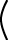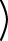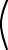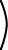No. For example there is no matrix 0-1 such that 00-1 = I

# Non-singular

In fact, it is worse than that. Many N × N matrices do not have an inverse. A matrix that does have an inverse is called non-singular. A matrix that does not is called singular. If the matrix A is non-singular, then:

AA-1 = A-1A = I

A non-singular matrix has a corresponding inverse. A singular matrix is all alone; it has no inverse.

Here is a matrix that has an inverse:

A A-1 = I```
1   2
0   1

``````
1  -2
0   1

```=```
1  0
0  1

```A-1 A = I```
1  -2
0   1

``````
1   2
0   1

```=```
1  0
0  1

```Now, say that``` 1 2 0 1 ```p =``` 5 2 ```### QUESTION 11:

What is p? Use A-1 from above.